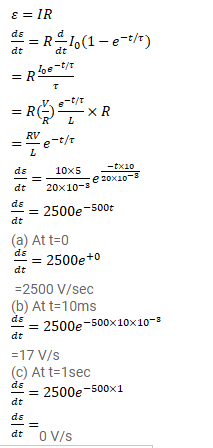# An inductor-coil of inductance 20mH having resistance 10Ω

Question:

An inductor-coil of inductance $20 \mathrm{mH}$ having resistance $10 \Omega$ is joined to an ideal battery of emf $5.0 \mathrm{~V}$. Find the rate of change of the induced emf at

(a) $t=0$,

(b) $t=10 \mathrm{~ms}$, and

(c) $t=1.0 \mathrm{~s}$.

Solution: